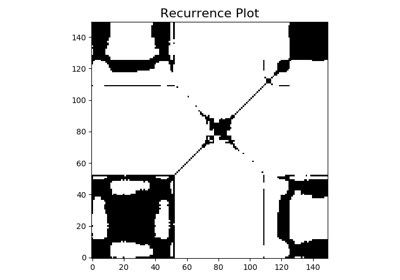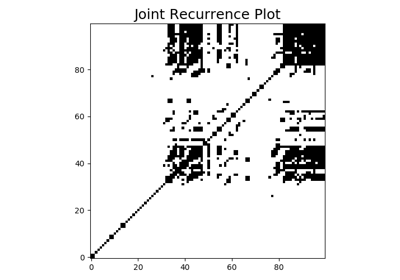# pyts.image.RecurrencePlot¶

class pyts.image.RecurrencePlot(dimension=1, time_delay=1, threshold=None, percentage=10, flatten=False)[source]

Recurrence Plot.

A recurrence plot is an image representing the distances between trajectories extracted from the original time series.

Parameters: dimension : int or float (default = 1) Dimension of the trajectory. If float, If float, it represents a percentage of the size of each time series and must be between 0 and 1. time_delay : int or float (default = 1) Time gap between two back-to-back points of the trajectory. If float, If float, it represents a percentage of the size of each time series and must be between 0 and 1. threshold : float, ‘point’, ‘distance’ or None (default = None) Threshold for the minimum distance. If None, the recurrence plots are not binarized. If ‘point’, the threshold is computed such as percentage percents of the points are smaller than the threshold. If ‘distance’, the threshold is computed as the percentage of the maximum distance. percentage : int or float (default = 10) Percentage of black points if threshold='point' or percentage of maximum distance for threshold if threshold='distance'. Ignored if threshold is a float or None. flatten : bool (default = False) If True, images are flattened to be one-dimensional.

Notes

Given a time series, the extracted trajectories arewhereis the dimension of the trajectories andis the time_delay. The recurrence plot, denoted, is the pairwise distance between the trajectorieswhereis the Heaviside function andis the threshold.

References

 [Rc0c3bc29e8b8-1] J.-P Eckmann, S. Oliffson Kamphorst and D Ruelle, “Recurrence Plots of Dynamical Systems”. Europhysics Letters (1987).

Examples

>>> from pyts.datasets import load_gunpoint
>>> from pyts.image import RecurrencePlot
>>> X, _, _, _ = load_gunpoint(return_X_y=True)
>>> transformer = RecurrencePlot()
>>> X_new = transformer.transform(X)
>>> X_new.shape
(50, 150, 150)


Methods

 __init__(self[, dimension, time_delay, …]) Initialize self. fit(self[, X, y]) Pass. fit_transform(self, X[, y]) Fit to data, then transform it. get_params(self[, deep]) Get parameters for this estimator. set_params(self, \*\*params) Set the parameters of this estimator. transform(self, X) Transform each time series into a recurrence plot.
__init__(self, dimension=1, time_delay=1, threshold=None, percentage=10, flatten=False)[source]

Initialize self. See help(type(self)) for accurate signature.

fit(self, X=None, y=None)[source]

Pass.

Parameters: X Ignored y Ignored self : object
fit_transform(self, X, y=None, **fit_params)

Fit to data, then transform it.

Fits transformer to X and y with optional parameters fit_params and returns a transformed version of X.

Parameters: X : numpy array of shape [n_samples, n_features] Training set. y : numpy array of shape [n_samples] Target values. **fit_params : dict Additional fit parameters. X_new : numpy array of shape [n_samples, n_features_new] Transformed array.
get_params(self, deep=True)

Get parameters for this estimator.

Parameters: deep : bool, default=True If True, will return the parameters for this estimator and contained subobjects that are estimators. params : mapping of string to any Parameter names mapped to their values.
set_params(self, **params)

Set the parameters of this estimator.

The method works on simple estimators as well as on nested objects (such as pipelines). The latter have parameters of the form <component>__<parameter> so that it’s possible to update each component of a nested object.

Parameters: **params : dict Estimator parameters. self : object Estimator instance.
transform(self, X)[source]

Transform each time series into a recurrence plot.

Parameters: X : array-like, shape = (n_samples, n_timestamps) X_new : array, shape = (n_samples, image_size, image_size) Recurrence plots. image_size is the number of trajectories and is equal to n_timestamps - (dimension - 1) * time_delay. If flatten=True, the shape is (n_samples, image_size * image_size).

## Examples using pyts.image.RecurrencePlot¶Recurrence PlotJoint Recurrence Plot